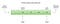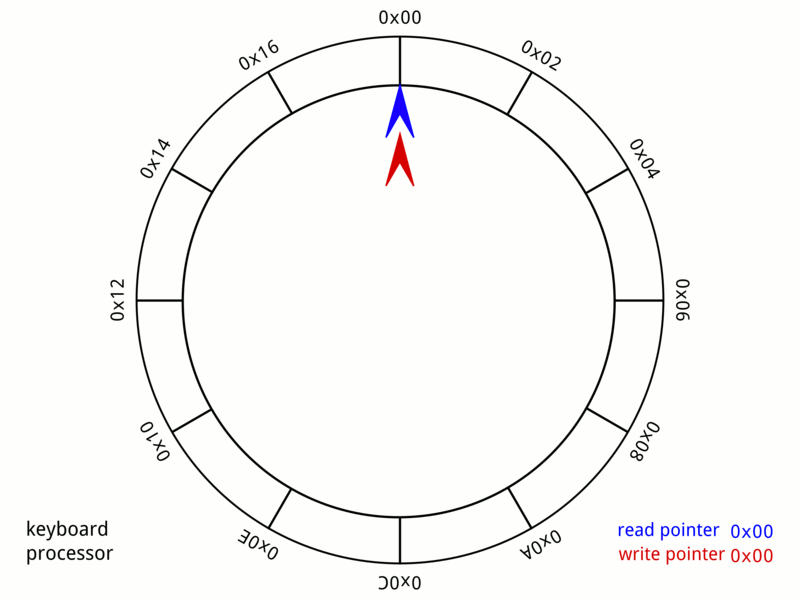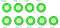# Queue in Javascript: How to create Priority, and Circular Queue

## Priority Queueimage by dailycodebuffer post
`class PriorityQueue {  #list = [];  #capacity;constructor(capacity) {    this.#capacity = Math.max(Number(capacity), 0) || null;  }get size() {    return this.#list.length;  }get isFull() {    return this.#capacity !== null && this.size === this.#capacity;  }get isEmpty() {    return this.size === 0;  }}`
`enqueue(item, priority = 0) {  priority = Math.max(Number(priority), 0);  const element = { item, priority };if (    this.isEmpty ||    element.priority <= this.#list[this.size - 1].priority  ) {    this.#list.push(element);  } else {    for (let index in this.#list) {      if (element.priority > this.#list[index].priority) {        this.#list.splice(index, 0, element);        break;      }    }  }return this.size;}`
`dequeue() {  return this.isEmpty ? null : this.#list.shift().item;}`
`class PriorityQueue {  #firstItem = null;  #lastItem = null;  #size = 0;  #capacity = null;    constructor(capacity = null) {    this.#capacity = capacity;  }get size() {    return this.#size;  }get isEmpty() {    return this.size === 0;  }get isFull() {    return this.#capacity ? this.size === this.#capacity : false;  }}`
`#createItem(value, priority) {  return {    next: null,    value,    priority  }}`
`enqueue(item, priority = 0) {  priority = Math.max(Number(priority), 0);if(!this.isFull) {    const newItem = this.#createItem(item, priority);(1) if(this.isEmpty) {       this.#firstItem = newItem;      this.#lastItem = newItem;    (2) } else if(newItem.priority <= this.#lastItem.priority) {      this.#lastItem.next = newItem;      this.#lastItem = newItem;    (3) } else if(newItem.priority > this.#firstItem.priority) {      newItem.next = this.#firstItem;      this.#firstItem = newItem;    (4) } else {      let current = this.#firstItem;while(current) {        if(newItem.priority > current.next.priority) {          newItem.next = current.next;          current.next = newItem;          break;        }        current = current.next;      }    }this.#size += 1;  }return this.size;}`
`dequeue() {  let removedItem = null;if(!this.isEmpty) {    removedItem = this.#firstItem.value;    this.#firstItem = this.#firstItem.next;    this.#size -= 1;  }return removedItem;}`

## Circular QueueRing Buffer — A 24-byte keyboard circular buffer. When the write pointer is about to reach the read pointer — because the microprocessor is not responding — the buffer stops recording keystrokes. On some computers, a beep would be playedimage by geeksforgeeks
`class CircularQueue {  #list = [];  #capacity;  #tail = -1;  #head = -1;  #size = 0;constructor(capacity = 10) {    this.#capacity = Math.max(Number(capacity), 0) || 10;  }get size() {    return this.#size;  }get isFull() {    return this.#size === this.#capacity;  }get isEmpty() {    return this.size === 0;  }}`
`enqueue(item) {  if (!this.isFull) {    this.#tail = (this.#tail + 1) % this.#capacity;    this.#list[this.#tail] = item;    this.#size += 1;        // queue was empty before this insertion    if (this.#head === -1) {      this.#head = this.#tail;    }  }return this.size;}`
`// tails starts at -1// than after the first increment// becomes 0 so if capacity is 30 % 3 // 01 % 3 // 12 % 3 // 23 % 3 // 0`
`dequeue() {  let item = null;if (!this.isEmpty) {    item = this.#list[this.#head];    delete this.#list[this.#head];    this.#head = (this.#head + 1) % this.#capacity;    this.#size -= 1;// reset tail and head if empty    if (!this.size) {      this.#head = -1;      this.#tail = -1;    }  }    return item;}`

## Conclusion

Blog & YouTube Channel for Web, UI & Software Development - beforesemicolon.comyoutube.com/c/BeforeSemicolon — Writer: Elson Correia

## More from Before Semicolon

Blog & YouTube Channel for Web, UI & Software Development - beforesemicolon.com — youtube.com/c/BeforeSemicolon — Writer: Elson Correia